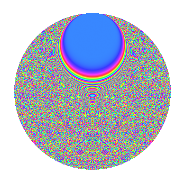# Properties

 Label 2100.2.ddLevel 2100 Weight 2 Character orbit dd Rep. character $$\chi_{2100}(11,\cdot)$$ Character field $$\Q(\zeta_{30})$$ Dimension 3776 Sturm bound 960

# Related objects

## Defining parameters

 Level: $$N$$ $$=$$ $$2100 = 2^{2} \cdot 3 \cdot 5^{2} \cdot 7$$ Weight: $$k$$ $$=$$ $$2$$ Character orbit: $$[\chi]$$ $$=$$ 2100.dd (of order $$30$$ and degree $$8$$) Character conductor: $$\operatorname{cond}(\chi)$$ $$=$$ $$2100$$ Character field: $$\Q(\zeta_{30})$$ Sturm bound: $$960$$

## Dimensions

The following table gives the dimensions of various subspaces of $$M_{2}(2100, [\chi])$$.

Total New Old
Modular forms 3904 3904 0
Cusp forms 3776 3776 0
Eisenstein series 128 128 0

## Trace form

 $$3776q - 6q^{4} - 20q^{6} - 6q^{9} + O(q^{10})$$ $$3776q - 6q^{4} - 20q^{6} - 6q^{9} + 9q^{12} - 48q^{13} - 6q^{16} - 30q^{21} - 40q^{22} - 2q^{24} - 12q^{25} - 68q^{28} + 9q^{30} - 30q^{33} + 24q^{34} + 32q^{36} - 12q^{37} - 44q^{40} + 28q^{42} + 12q^{45} - 42q^{46} - 56q^{49} + 70q^{52} + q^{54} - 72q^{57} + 38q^{58} + 45q^{60} - 12q^{61} + 24q^{64} + 11q^{66} - 48q^{69} - 12q^{70} - 39q^{72} + 4q^{73} - 128q^{76} + 122q^{78} + 18q^{81} + 92q^{82} + 9q^{84} - 40q^{85} - 10q^{88} + 6q^{90} + 8q^{93} - 22q^{94} + 15q^{96} + 72q^{97} + O(q^{100})$$

## Decomposition of $$S_{2}^{\mathrm{new}}(2100, [\chi])$$ into newform subspaces

The newforms in this space have not yet been added to the LMFDB.

## Hecke characteristic polynomials

There are no characteristic polynomials of Hecke operators in the database